# Stochastic quantization of the Euclidean $$\Phi^4_3$$ quantum field theory

Lectures organised in the context of the graduate school of the University of Milan.

Lecturer. Massimiliano Gubinelli

Schedule. February 15, 16, 18, 22, 25. 10–12 and 14–16. Online via Zoom. (Meeting ID: 946 4276 2037 / Passcode: 954150) (link)

The goal of Euclidean quantum field theory (see, e.g. [4,2]) is to build probability measures on the space of distributions on $$\mathbb{R}^n$$ satisfying properties such as Euclidean invariance, reflection positivity and non-triviality, that allows to recover an interacting relativistic quantum field satisfying Wightman axioms. Stochastic quantization, first proposed by Parisi–Wu and Nelson, is a method of construction of such measures via stationary solutions of a stochastic partial differential equations driven by additive Gaussian white noise (for a non–rigorous introduction see ).

In this course we will learn about the stochastic quantization of the Euclidean quantum field theory of a scalar boson with quartic interaction on $$\mathbb{R}^3$$ and its main properties. We follow the proof in  which builds the $$\Phi^4_3$$ measure as the limit of the invariant measure of a finite dimensional system of stochastic differential equations. The proof proposed uses several analytic and probabilistic techniques, such as white noise analysis, weighted Besov spaces on lattice and paraproducts, which find also applications in other problems arising in the study of deterministic and stochastic singular differential equations. All these tools and ideas will be gradually introduced and explained during the lectures. The course is as much as possible self-contained and requires as prerequisite only basic knowledge of stochastic and functional analysis.

### Outline

• An introduction to Euclidean quantum field theories: Euclidean invariance, reflection positivity, singularities.

• Stochastic differential equations and their invariant measures. Langevin dynamics. Stochastic quantisation (SQ).

• Problems of SQ. Local singularities and infinite volume limit. Need of renormalization and coercivity.

• The local structure of the $$\Phi^4_3$$ dynamics, expansion, paracontrolled analysis. Local solutions of the renormalized equation.

• The large scale structure of the renormalized equation. Tightness estimates.

• Existence of the infinite volume measure and some of its properties: non-Gaussianity

### Bibliography

 P. H. Damgaard and H. Hüffel, editors. Stochastic quantization. World Scientific Publishing Co., Inc., Teaneck, NJ, 1988.

 James Glimm and Arthur Jaffe. Quantum physics. Springer-Verlag, New York, Second edition, 1987. A functional integral point of view.

 M. Gubinelli and M. Hofmanová. A PDE construction of the euclidean $$\Phi^4_3$$ quantum field theory. ArXiv preprint arXiv:1810.01700, 2018.

 Barry Simon. The $$P (\phi)_2$$ Euclidean (quantum) field theory. Princeton University Press, Princeton, N.J., 1974. Princeton Series in Physics.

## Extended lecture notes

• First part: from QM to QFT (notes)
• Second part: stochastic quantization of $$\Phi^4_3$$ (notes)

## Journal and scripts of the lectures

• Monday   15/2, 10-12 : Lecture 1. Introduction. Quantum Mechanics. GNS representation. (script)
• Monday   15/2, 14-16 : Lecture 2. Wightman functions. Schwinger functions. Gaussian and Markovian RP processes. (script)
• Tuesday  16/2, 10-12 : Lecture 3. OU process, reconstruction of QM. Perturbations of RP processes. (script)
• Tuesday  16/2, 14-16 : Lecture 4. RP perturbation of Markov processes. Euclidean QFT. (script
• Thursday 18/2, 10-12 : Lecture 5. Interactions. Problems. Stochastic quantization in finite dimension. (script
• Thursday 18/2, 14-16 : Lecture 6. Langevin dynamics. Estimates. Infinite volume limit.  (script)
• Monday   22/2, 10-12 : Lecture 7. Small scale limit. Divergences and renormalization. Besov spaces and paraproducts. Apriori estimates in two dimensions. (script)
• Monday   22/2, 14-16 : Lecture 8. Tightness in two dimensions. Estimates in three dimensions. Paracontrolled expansion. (script)
• Thursday 25/2, 10-12 : Lecture 9. The second renormalization, commutator lemmas. (script)
• Thursday 25/2, 14-16 : Lecture 10. Tightness in three dimensions and infinite volume limit. Some properties of the measure.  (script)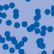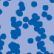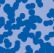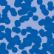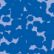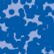## News

Florian Schweiger erhielt den Hausdorff-Gedächtnispreis 2021 der Fachgruppe Mathematik für die beste Dissertation. Er fertigte die Dissertation unter der Betreuung von Prof. Stefan Müller an. Unter anderen wurde Vanessa Ryborz mit einem Preis der Bonner Mathematischen Gesellschaft für ihre von Prof. Sergio Conti betreute Bachelorarbeit ausgezeichnet. (18.01.2022)

Prof. Dr. Sergio Albeverio has been elected into the Academia Europaea and the Accademia Nazionale dei Lincei (more; 02.12.2021).

Der SFB 1060 Die Mathematik der emergenten Effekte hat eine dritte Förderperiode erhalten. (26.11.20)

Prof. Dr. Andreas Eberle erhält den diesjährigen Lehrpreis der Universität Bonn. (22.07.2020)

Herr Dr. Richard Höfer erhielt den Hausdorff-Gedächtnispreis 2019 der Fachgruppe Mathematik für die beste Disseration. Betreut wurde die Arbeit von Prof. J. Velázquez (29.01.2020).

Contact

Managing Director: Prof. Dr. Massimiliano Gubinelli